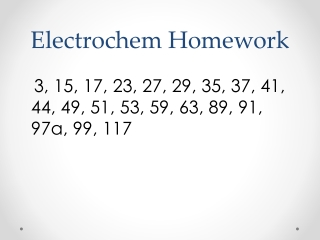DownloadDownload PresentationElectrochem Homework

# Electrochem Homework

Download Presentation## Electrochem Homework

- - - - - - - - - - - - - - - - - - - - - - - - - - - E N D - - - - - - - - - - - - - - - - - - - - - - - - - - -
##### Presentation Transcript

1. Electrochem Homework 3, 15, 17, 23, 27, 29, 35, 37, 41, 44, 49, 51, 53, 59, 63, 89, 91, 97a, 99, 117

2. Oxidation Numbers • # of e- gained or lost when forming a compound • If ion, actual charge • Use the Periodic Table!

3. General Rules • Free uncombined state of atoms -> 0 Ex. Na, K, S8 , Cl2 • Sum of oxidation #’s in neutral compound is zero • Polyatomic ions, oxidation #’s add up to the charge on the ion • F has a -1 ox. state

4. General Rules Continued • H usually has an ox. state of +1 Exception: NaH where H is -1 • O usually has an ox. State of -2 Exceptions: O-1 and O-1/2 • Use the Periodic Table to find the location of the element

5. Examples • HNO3 • NO3-1

6. New Method # of valence electrons - # of unshared electrons Midway number - # of bonding electrons assigned to it* oxidation number * Higher electronegative atom gets the e-

7. Examples • NH3 • CH3OH

8. Why do we need a new method? • SO4-2 vs. S2O3-2

9. Electrochemistry • Study of the relationship between chemical change and electrical work • Application of thermodynamics in everyday applications Ex. Radios, iPods, calculators • Battery houses a spontaneous chemical reaction that releases free energy to produce electricity

10. Electrochemistry cont. • 2nd type of reaction: Nonspontaneous and absorbs free energy from an external source of electricity Ex. Electroplating, recovery of metals from ores

11. Electrochemistry cont. • Amount of energy (electrical) consumed or produced in chemical reactions can be accurately measured • All electrochemical reactions involve the transfer of electrons Redox reactions! • The electrochemical cell contains the reacting system

12. Electrochemistry cont. • Sites of oxidation and reduction separated physically so that reduction occurs at one electrode and oxidation occurs at the other electrode Red Cat and An Ox

13. Examples of Redox reactions 4 Al(s) + 3 O2(g) ---> 2 Al2O3(s)

14. Balancing redox reactions • Write the unbalanced equation • Assign oxidation numbers • Choose coefficients that make the transfer of electrons the same • Balance the charge • Balance remaining elements if necessary

15. Balancing In Acidic Media • Add H+ and H2O to balance charge Ex. Cr2O7-2 + I- Cr+3 + IO3-

16. Balancing In Basic Media • Balance charge by adding OH- and H2O Ex. Cr2O7-2 + I- Cr+3 + IO3-

17. Balance in Acid • MnO4- + Br- MnO2 + BrO2-1

18. Balance in Base (Drano) • Al + H2O [Al(OH)4]-+ H2

19. Balance in Acid Cu + NO3- Cu+2 + NO

20. Half Rxn Method for balancing redox equations • Overall reaction split into two half reactions • Separates oxidation and reduction half reactions • Easy to balance redox reactions occurring in acidic or basic solutions

21. How? 1. Divide equation into two half reactions 2. Balance the atoms and charges in each half reaction - e- added to left is reduction - e- added to right is oxidation 3. Multiply by integer so e- lost = e- gained 4. Add balanced equations

22. Ex. Balance in acidic media Ex. Cr2O7-2 + I- Cr+3 + I2

23. Ex. Balance in basic media MnO4-1 + C2O4-2 MnO2 + CO3-2

24. Breathalyzer reaction Cr2O7-2+ C2H5OH + H+ Cr+3 + C2H4O + H2O

25. Equivalents • Acids and Bases  Just deal with the number of H+ or OH- being donated Ex. HCl -> 1 equivalent H3PO4 -> 3 equivalents NaOH -> 1 equivalent Ca(OH)2 -> 2 equivalents

26. Acid Reaction H2SO4 + H2O 2 H+ + SO4-2 1 mol 2 mol 1 mol 98.0 g/mol 2.0 g/mol 96.0 g/mol 2 equivalents 1 equivalent HNO3 – 63.01 g 1 equiv. CH3COOH – 60.03 g 1 equiv. H2SO4 - 49.04 g 1 equivalent of Ca(OH)2 - 37.05 g

27. Normality • Molarity = moles of solute Liters of solution • Normality = equivalents of solute Liters of solution • N = M x #equivalents

28. Equivalents and Redox • Not only do equivalents pertain to # H+ or # OH- but also to electrons • Equivalent – mass of oxidizing or reducing substance that gains or loses 1 mole of electrons • 1 mole of electrons equals 1 equivalent of electrons

29. Equivalents • One equiv. oxidized reacts with one equiv. reduced 1 eq. ox. = 1 eq. red. • If redox equation is balanced – mole ratios can be used in stoichiometry • If NOT balanced – equivalents can be used

30. END!